Smartick is an advanced online program that teaches kids math and coding in only 15 min. a day

Mar08

# Learn How to Do Single Digit Division

Today, we are going to take a look at how to do single digit division.

The first thing we need to remember is the vocabulary of the components of division:

• The dividend is the number that is going to be divided.
• The divisor is the number that the dividend is divided by.
• The quotient is the result of the division,
• The remainder is the leftover quantity.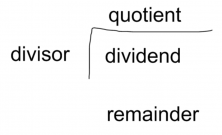### Single Digit Division

Exercise #1:

Divide 1728 by 6.

STEP 1: Put 1728 in the dividend position, and the 6 in place of the divisor.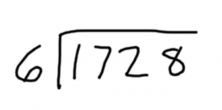STEP 2: Take the first digit of the dividend, in this case, 1. Since 1 is smaller than the divisor, 6, we cannot divide it.  Therefore, we have to take the following digit of the dividend, which is 7.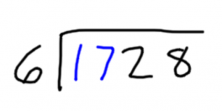STEP 3: We are searching for a number, which, when multiplied by 6, gives us 17. 2×6=12, which is less than 17, but 3×6=18, which is greater than 17. We need to take the number that is the closest, without going over, which in this case is 2.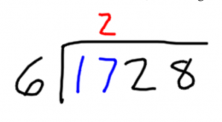STEP 4: Put the answer of 6 x 2 beneath the dividend and subtract it from the first two digits.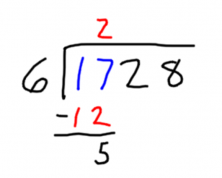STEP 5: The next step is to bring down the next digit of the dividend, which is the 2. Thus, the number that we are left with is 52. We are searching for a number, which, when multiplied by 6, gives us 52. 6 x 8 = 48, but 6 x 9 = 54. Since we are not able to go over 52, we take the number 8 and do the same as in step 4: 52 – 48 = 4.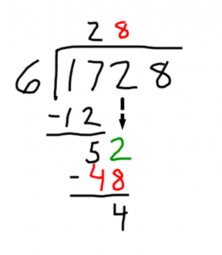STEP 6:  We repeat step 5 with the next digit of the dividend, which is 8. Now, we have to search for a number, which, when multiplied by 6, gives us 48. We already know it! It’s 8. 48 – 48 = 0. Since we are not left with any digits of the dividend to drop down, we are finished. The quotient of this single-digit division is 288 and the remainder is 0.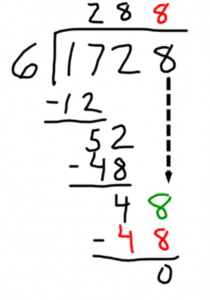Now you have seen an example of how to do single digit division! To learn even more, check out similar exercises:

More Exercises with 1 Digit Division

Practice 2 and 3 Digit Division

What did you think of this post? Did we help you with division? If so, share it with your friends, so they can also review! And don’t forget that on Smartick you will be able to learn to do division and much more elementary math!